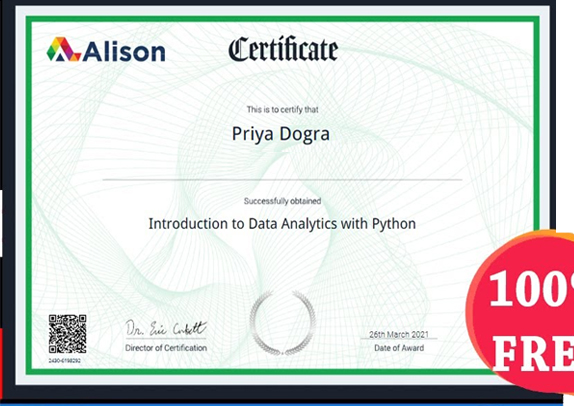Home Certification Introduction to Data Analytics with Python Assessment Answers – Alison

# Introduction to Data Analytics with Python Assessment Answers – Alison

0## Enroll Here: Introduction to Data Analytics with Python Free Certification

Question 1: If X and Y are independent events, then ______________. Choose two.

• the occurrence of X affects the occurrence of Y
• the occurrence of X does not affect the occurrence of Y
• the occurrence of Y affects the occurrence of X
• the occurrence of Y does not affect the occurrence of X

Question 2: Which of the following method of assigning probabilities is based on historical data? Choose one.

• Subjective probabilty
• Relative frequency probability
• Classical method
• Objective probablity

Question 3: True or False. A conditional probability is the probability of one event, given that another event has occurred.

• True
• False

Question 4: The most frequently occurring value in a data set is called the _________ of the data set. Fill in the blank.

Question 5: True or False. Stratified random sampling is a method of selecting a sample in which the population is divided into strata, and then random samples are drawn from each stratum

• True
• False

Question 6: True or False. Probability is the numerical likelihood that an event will occur.

• True
• False

Question 7: True or False. Coefficient of variation is the ratio of the standard deviation to the mean, generally expressed as a percentage.

• True
• False

Question 8: Which of the following is a type of sampling distribution? Choose one.

• Sample distribution of sample standard deviation
• Sample distribution of sample median
• Sample distribution of sample mode
• Sample distribution of sample proportion

Question 9: Consider the following data function in Python programming:

def x(data)

Z = max(data) – min(data)

return Z

What will the defined data function above calculate? Choose one.

• Median
• Mode
• Range
• Variance

Question 10: Median is not applicable to __________ data type. Fill in the blank.

Question 11: True or False. There are three methods of assigning probabilities.

• True
• False

Question 12: True or False. An independent event is always mutually exclusive.

• True
• False

Question 13: True or False. If two groups of numbers have the same mean, then their medians must also be equal.

• True
• False

Question 14: True or False. Pie charts are used for the visualization of categorical data.

• True
• False

Question 15: True or False. A mutually exclusive event will always be an independent event.

• True
• False

Question 16: In skewed data, the best measure of central tendency is the __________. Fill in the blank.

Question 17: If P = 0.4 and n = 200, what is P(0.40 ≤ p ≤ 0.45)? Choose one.

• 0.354
• O.4251
• 0.4
• 0.532

Question 18: True or False. Stratified random sampling is a method of selecting a sample in which various strata are selected from the sample.

• True
• False

Question 19: True or False. It is not impossible to find the median of an odd-numbered data set.

• True
• False

Question 20: True or False. Frequency polygons and bar charts are used for continuous data.

• True
• False

Question 21: The 50th percentile of a normally distributed data set will represent its __________. Choose two.

• Sum
• Range
• Mode
• Mean
• Median

Question 22: True or False. In skewed data, the best measure of central tendency is the Mean.

• True
• False

Question 23: ______________ probabily comes from a person’s intuition or reasoning. Fill in the blank.

Question 24: Which Python command is used for accessing the second row of an excel file named “data file” having only two rows? Choose one.

• datafile.tail()
• datafile.loc
• datafile.loc
• datafile.iloc

Question 25: The Median is applicable for which of the following data types? Choose three.

• Cardinal
• Interval
• Nominal
• Ordinal
• Ratio

Question 26: True or False. To get the 3rd, 4th and 5th row of a datafile “df” in Python, the programmer can write: df.loc[2,3,4]

• True
• False

Question 27: If the true proportion of customers below 20 years is given by P = 0.35, what is the probability that a sample size of 100 yields a sample proportion between 0.3 and 0.4? Choose one.

• 0.53
• 0.706
• 0.961
• 0.827

Question 28: Some CDs produced by a manufacturer are defective. From the production line, 5 CDs are selected and inspected. How many sample points exist in this experiment? Choose one.

• 32
• 30
• 10
• 25

Question 29: Percentiles are measures of central tendency that divide a group of data into ________ parts. Answer in numeric form.